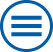## The group contributes to the following mathematical research topics of WIAS:

Analysis of Partial Differential Equations and Evolutionary Equations

Partial differential equations form an adequate and powerful instrument to provide a mathematical model for nature. At the Weierstrass Institute this research has three essential focuses: (a) Rigorous mathematical analysis of general evolution equations in terms of existence, uniqueness and regularity of different types of solutions, (b) Development of variational approaches using the toolbox of the calculus of variations, (c) Regularity results for solutions of elliptic and parabolic partial differential equations. [>> more]

Analysis of ordinary and partial stochastic diff erential equations

An ordinary differential equation is often used to model the movement of a particle. Similarly, partial differential equation can be used to describe the evolution of a total of trajectories of particles. It is natural to add randomness to such models: sometimes because this is a more realistic description which takes into account random noise, sometimes because this randomness is fundamental to the model itself as is the case for financial markets. [>> more]

Direct and inverse problems for the Maxwell equations

The work is focussed on models for inductive heating of steel and for light scattering by periodic surface structures. For this the quasi-stationary Maxwell equation is coupled with nonlinear partial differential equations and the timeharmonic Maxwell equation is combined with special radiation conditions, respectively. The convergence of numerical methods and several inverse promblems are analyzed. [>> more]

Free boundary problems for partial differential equations

Free boundary problems for partial differential equation describe problems such that a partial differential equation is considered on a domain depending on the solution to the equation. Free boundary problems are investigated in connection with energy technology, for example. [>> more]

Hysteresis operators and rate-independent systems

Time-dependent processes in physics, biology, and economics often exhibit a rate-independent input-output behavior. Quite often, such processes are accompanied by the occurrence of hysteresis phenomena induced by inherent memory effects. There are two methods to describe such processes. 1. In order to describe the current state, one can add internal variables to the observable variables, and describes the evolution of internal variables. 2. For many cases one can find hysteresis operators directly describing the input-output-behavior, such that the state depends on the history of the considered process. [>> more]

Modeling, analysis and numerics of phase field models

A diffuse phase field model is a mathematical model for describing microstructural phenomena and for predicting morphological evolution on the mesoscale. It is applied to a wide variety of material processes such as solidification, coarsening in alloys, crack propagation and martensitic transformations. [>> more]

Multi-scale modeling, asymptotic analysis, and hybrid models

To understand the interplay between different physical effects one often needs to consider models involving several length scales. The aim in this mathematical topic is the derivation of effective models for the efficient description of the processes. The understanding of the transfer between different scales relies on mathematical methods such as homogenization, asymptotic analysis, or Gamma convergence. The generated effective models are coupled partial differential equations combining volume and interfacial effects. [>> more]

Nonlinear kinetic equations

Kinetic equations describe the rate at which a system or mixture changes its chemical properties. Such equations are often non-linear, because interactions in the material are complex and the speed of change is dependent on the system size as well as the strength of the external influences. [>> more]

Systems of partial differential equations: modeling, numerical analysis and simulation

The mathematical modelling of many scientific and technological problems leads to (initial) boundary value problems with systems of partial differential equations (PDEs). [>> more]

## Archive

### Further mathematical research topics where the institute has expertise in:

Magnetohydrodynamics

For the production of semiconductor crystals, electromagnetic fields are often used to produce heat by induction. Moreover, Lorentz forces can improve the melt motion during crystal growth processes. Their modeling leads to a system of coupled partial differential equations. [>> more]

Plates, Beams, Shells and Arches

An efficient description of the mechanical behavior of special 3D bodies, whose dimensions in one or two directions are small in comparison to the other dimensions, is possible with so-called plate models or beam models. [>> more]